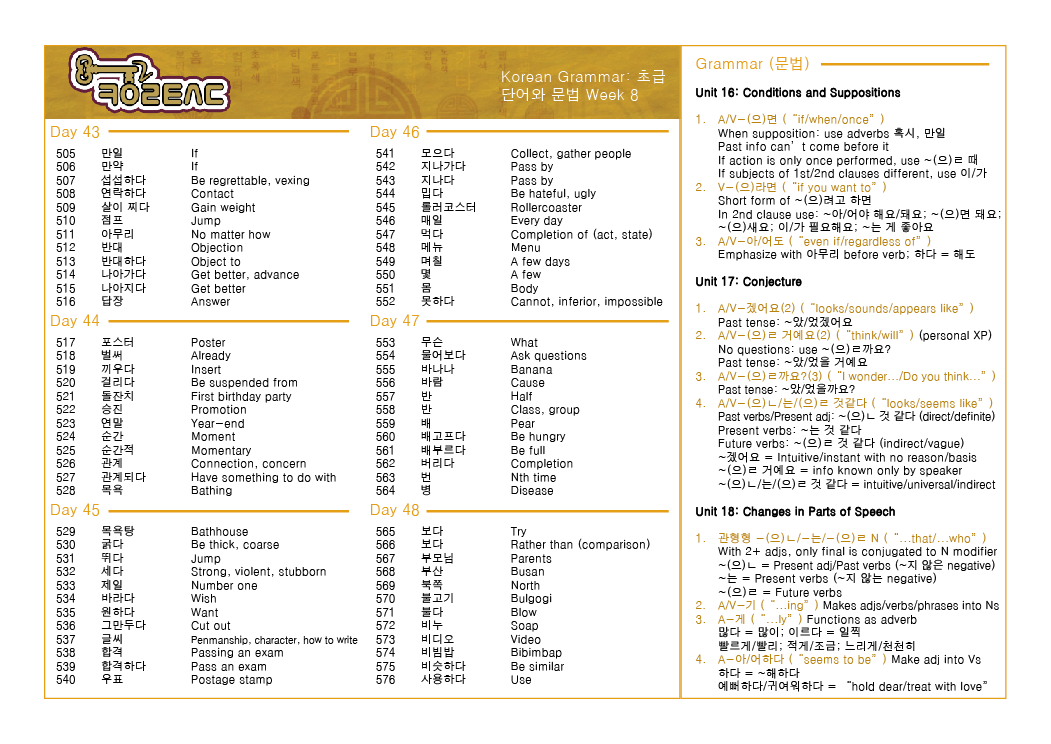Open Sidebar

# Low Intermediate Week 8 Review## Most Important Things We Went Over This Week

• V~(으)려면 = short form of V~(으)려고 하면
• V~(으)려면 often uses ~아/어야 해요/돼요, ~(으)면 돼요, ~(으)세요, 이/가 필요해요, ~는 게 좋아요 in the second clause
• A/V~(으)면 = if/when, A/V~아/어도 = even if (use 아무리 to express “no matter how much”)
• A/V~(으)ㄹ까요 = “Do you think…?/I wonder…” and is often followed by:
• ~(으)ㄹ 거예요 = supposition known only by the speaker
• ~(으)ㄴ/는/(으)ㄹ 것 같다 = supposition based on intuitive, subjective reasons – can be in generic (ALL) cases even regardless of reasons
• ~겠어요 = supposition based on intuition – instantaneous and has no reasons
• ~(으)ㄴ = past (also direct), also adj /는 = present /(으)ㄹ = future (also vague) 것 같다
• 관형형 ~(으)ㄴ = past, also adj /~는 = present /~(으)ㄹ = future N (Noun modifiers) – only FINAL adj is conjugated if more than one
• ~기 = “~ing” vs ~게 = “~ly”
• A~아/어하다 = “seems to be”

## Things that Need More Conversation Practice

• A/V~(으)면 vs. V~(으)려면
•  V~(으)려면 with all the different endings
• A/V~(으)ㄹ까요 with answers: ~(으)ㄹ 거예요, ~(으)ㄴ/는/(으)ㄹ 것 같다, ~겠어요
• Past/present/future noun modifiers (~(으)ㄴ/~는/~(으)ㄹ N) and suppositions (~(으)ㄴ/는/(으)ㄹ 것 같다)
• ~기 vs. ~게

## Things that Need Quizzed

• What’s the longer form of V~(으)려면?
• What should you use with A/V~아/어도 to emphasize it? (아무리)
• Difference between ~(으)ㄹ 거예요, ~(으)ㄴ/는/(으)ㄹ 것 같다, and ~겠어요.
• How can you tell which is past/present/future? (~(으)ㄴ/는/~(으)ㄹ)
• How can you make an adjective “seems to be”?